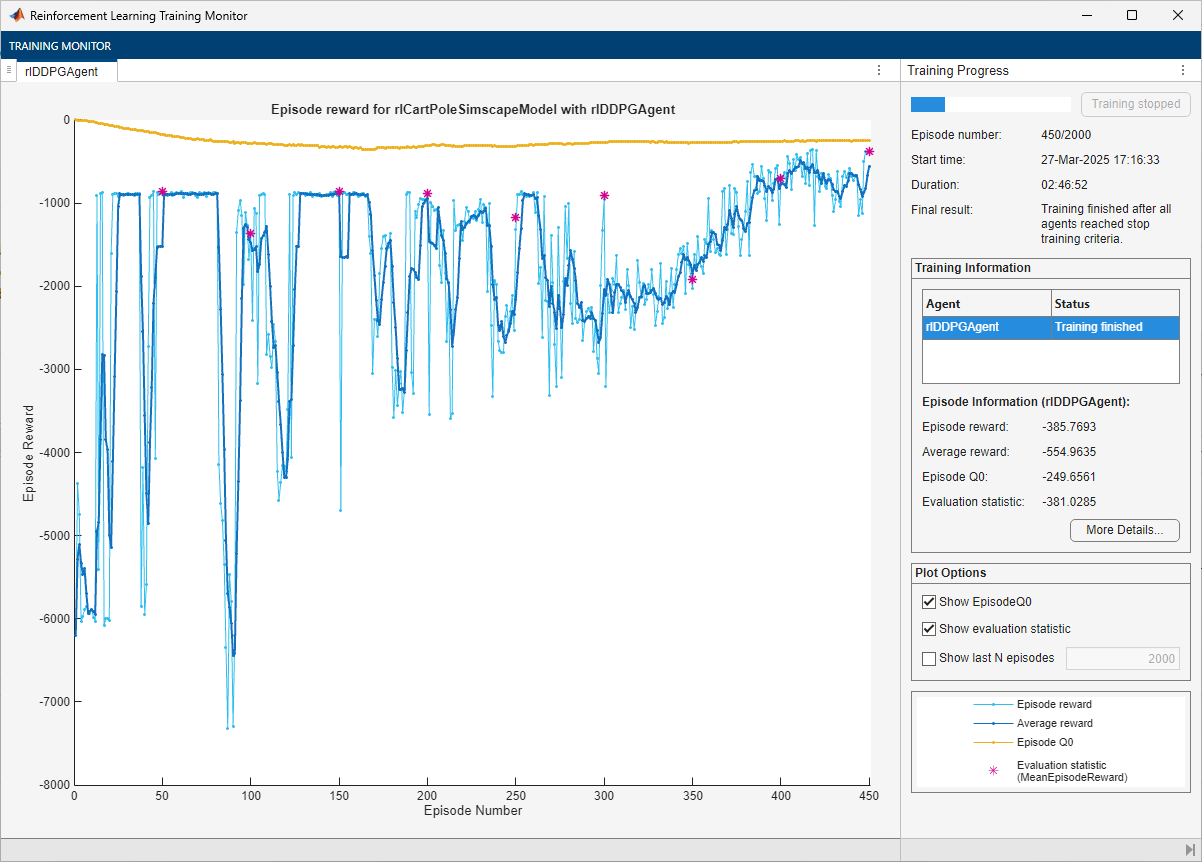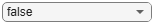# Train DDPG Agent to Swing Up and Balance Cart-Pole System

This example shows how to train a deep deterministic policy gradient (DDPG) agent to swing up and balance a cart-pole system modeled in Simscape™ Multibody™.

For more information on DDPG agents, see Deep Deterministic Policy Gradient (DDPG) Agents. For an example showing how to train a DDPG agent in MATLAB®, see Compare DDPG Agent to LQR Controller.

### Cart-Pole Simscape Model

The reinforcement learning environment for this example is a pole attached to an unactuated joint on a cart, which moves along a frictionless track. The training goal is to make the pole stand upright without falling over using minimal control effort.

Open the model.

```mdl = "rlCartPoleSimscapeModel"; open_system(mdl)```The cart-pole system is modeled using Simscape Multibody.For this model:

• The upward balanced pole position is 0 radians, and the downward hanging position is `pi` radians.

• The force action signal from the agent to the environment is from –15 to 15 N.

• The observations from the environment are the position and velocity of the cart, and the sine, cosine, and derivative of the pole angle.

• The episode terminates if the cart moves more than 3.5 m from the original position.

• The reward ${\mathit{r}}_{\mathit{t}}$, provided at every timestep, is

`${\mathbit{r}}_{\mathbit{t}}=\text{\hspace{0.17em}}-0.1\left(5{{\theta }_{\mathbit{t}}}^{2}\text{\hspace{0.17em}}+{{\mathit{x}}_{\mathbit{t}}}^{2}\text{\hspace{0.17em}}+\text{\hspace{0.17em}}0.05{\mathit{u}}_{\mathit{t}-1}^{2}\right)-100\mathit{B}$`

Here:

• ${\theta }_{\mathit{t}}$ is the angle of displacement from the upright position of the pole.

• ${\mathit{x}}_{\mathit{t}}$ is the position displacement from the center position of the cart.

• ${\mathit{u}}_{\mathit{t}-1}$ is the control effort from the previous time step.

• $\mathit{B}$ is a flag (1 or 0) that indicates whether the cart is out of bounds.

### Create Environment Interface

Create a predefined environment interface for the pole.

`env = rlPredefinedEnv("CartPoleSimscapeModel-Continuous")`
```env = SimulinkEnvWithAgent with properties: Model : rlCartPoleSimscapeModel AgentBlock : rlCartPoleSimscapeModel/RL Agent ResetFcn : [] UseFastRestart : on ```

The interface has a continuous action space where the agent can apply possible torque values from –15 to 15 N to the pole.

Obtain the observation and action information from the environment interface.

```obsInfo = getObservationInfo(env); actInfo = getActionInfo(env);```

Specify the simulation time `Tf` and the agent sample time `Ts` in seconds.

```Ts = 0.02; Tf = 25;```

Fix the random generator seed for reproducibility.

`rng(0)`

### Create DDPG Agent

DDPG agents use a parametrized Q-value function approximator to estimate the value of the policy. A Q-value function critic takes the current observation and an action as inputs and returns a single scalar as output (the estimated discounted cumulative long-term reward for which receives the action from the state corresponding to the current observation, and following the policy thereafter).

To model the parametrized Q-value function within the critic, use a neural network with two input layers (one for the observation channel, as specified by `obsInfo`, and the other for the action channel, as specified by `actInfo`) and one output layer (which returns the scalar value). Note that `prod(obsInfo.Dimension)` and `prod(actInfo.Dimension)` return the number of dimensions of the observation and action spaces, respectively, regardless of whether they are arranged as row vectors, column vectors, or matrices.

Define the network as an array of layer objects. Assign names to the input and output layers of each path. These names allow you to connect the paths and then later explicitly associate the network input and output layers with the appropriate environment channel.

For more information on creating a deep neural network value function representation, see Create Policies and Value Functions.

```% Define path for the state input statePath = [ featureInputLayer(prod(obsInfo.Dimension),Name="NetObsInLayer") fullyConnectedLayer(128) reluLayer fullyConnectedLayer(200,Name="sPathOut")]; % Define path for the action input actionPath = [ featureInputLayer(prod(actInfo.Dimension),Name="NetActInLayer") fullyConnectedLayer(200,Name="aPathOut",BiasLearnRateFactor=0)]; % Define path for the critic output (value) commonPath = [ additionLayer(2,Name="add") reluLayer fullyConnectedLayer(1,Name="CriticOutput")]; % Create layerGraph object and add layers criticNetwork = layerGraph(statePath); criticNetwork = addLayers(criticNetwork,actionPath); criticNetwork = addLayers(criticNetwork,commonPath); % Connect paths and convert to dlnetwork object criticNetwork = connectLayers(criticNetwork,"sPathOut","add/in1"); criticNetwork = connectLayers(criticNetwork,"aPathOut","add/in2"); criticNetwork = dlnetwork(criticNetwork);```

Display the number of weights and plot the network configuration.

`summary(criticNetwork)`
``` Initialized: true Number of learnables: 27.1k Inputs: 1 'NetObsInLayer' 5 features 2 'NetActInLayer' 1 features ```
`plot(criticNetwork)`Create the critic representation using the specified deep neural network and options. You must also specify the action and observation information for the critic, which you already obtained from the environment interface. For more information, see `rlQValueFunction`.

```critic = rlQValueFunction(criticNetwork, ... obsInfo,actInfo,... ObservationInputNames="NetObsInLayer", ... ActionInputNames="NetActInLayer");```

DDPG agents use a parametrized deterministic policy over continuous action spaces, which is learned by a continuous deterministic actor. This actor takes the current observation as input and returns as output an action that is a deterministic function of the observation.

To model the parametrized policy within the actor, use a neural network with one input layer (which receives the content of the environment observation channel, as specified by `obsInfo`) and one output layer (which returns the action to the environment action channel, as specified by `actInfo`).

Since the output of `tanhLayer` is limited between -1 and 1, scale the network output to the range of the action using `scalingLayer`.

```actorNetwork = [ featureInputLayer(prod(obsInfo.Dimension)) fullyConnectedLayer(128) reluLayer fullyConnectedLayer(200) reluLayer fullyConnectedLayer(prod(actInfo.Dimension)) tanhLayer scalingLayer(Scale=max(actInfo.UpperLimit)) ];```

Convert to dlnetwork and display the number of weights.

```actorNetwork = dlnetwork(actorNetwork); summary(actorNetwork)```
``` Initialized: true Number of learnables: 26.7k Inputs: 1 'input' 5 features ```

Create the actor in a similar manner to the critic. For more information, see `rlContinuousDeterministicActor`.

`actor = rlContinuousDeterministicActor(actorNetwork,obsInfo,actInfo);`

Specify training options for the critic and the actor using `rlOptimizerOptions`.

```criticOptions = rlOptimizerOptions(LearnRate=1e-03,GradientThreshold=1); actorOptions = rlOptimizerOptions(LearnRate=5e-04,GradientThreshold=1);```

Specify the DDPG agent options using `rlDDPGAgentOptions`, and include the training options for the actor and critic.

```agentOptions = rlDDPGAgentOptions(... SampleTime=Ts,... ActorOptimizerOptions=actorOptions,... CriticOptimizerOptions=criticOptions,... ExperienceBufferLength=1e6,... MiniBatchSize=128);```

You can also modify the agent options using dot notation.

```agentOptions.NoiseOptions.Variance = 0.4; agentOptions.NoiseOptions.VarianceDecayRate = 1e-5;```

Alternatively, you can also create the agent first, and then access its option object and modify the options using dot notation.

Then, create the agent using the actor, critic and agent options objects. For more information, see `rlDDPGAgent`.

`agent = rlDDPGAgent(actor,critic,agentOptions);`

### Train Agent

To train the agent, first specify the training options. For this example, use the following options.

• Run each training episode for at most 2000 episodes, with each episode lasting at most `ceil(Tf/Ts)` time steps.

• Display the training progress in the Episode Manager dialog box (set the `Plots` option) and disable the command line display (set the `Verbose` option to `false`).

• Stop training when the agent receives an average cumulative reward greater than –400 over five consecutive episodes. At this point, the agent can quickly balance the pole in the upright position using minimal control effort.

• Save a copy of the agent for each episode where the cumulative reward is greater than –400.

For more information, see `rlTrainingOptions`.

```maxepisodes = 2000; maxsteps = ceil(Tf/Ts); trainingOptions = rlTrainingOptions(... MaxEpisodes=maxepisodes,... MaxStepsPerEpisode=maxsteps,... ScoreAveragingWindowLength=5,... Verbose=false,... Plots="training-progress",... StopTrainingCriteria="AverageReward",... StopTrainingValue=-400,... SaveAgentCriteria="EpisodeReward",... SaveAgentValue=-400);```

Train the agent using the `train` function. Training this agent process is computationally intensive and takes several hours to complete. To save time while running this example, load a pretrained agent by setting `doTraining` to `false`. To train the agent yourself, set `doTraining` to `true`.

```doTraining = false; if doTraining % Train the agent. trainingStats = train(agent,env,trainingOptions); else % Load the pretrained agent for the example. load("SimscapeCartPoleDDPG.mat","agent") end```### Simulate DDPG Agent

To validate the performance of the trained agent, simulate it within the cart-pole environment. For more information on agent simulation, see `rlSimulationOptions` and `sim`.

```simOptions = rlSimulationOptions(MaxSteps=500); experience = sim(env,agent,simOptions);````bdclose(mdl)`